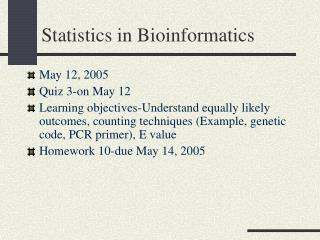Download PresentationStatistics in Bioinformatics

# Statistics in Bioinformatics - PowerPoint PPT PresentationDownload Presentation## Statistics in Bioinformatics

- - - - - - - - - - - - - - - - - - - - - - - - - - - E N D - - - - - - - - - - - - - - - - - - - - - - - - - - -
##### Presentation Transcript

1. Statistics in Bioinformatics • May 12, 2005 • Quiz 3-on May 12 • Learning objectives-Understand equally likely outcomes, counting techniques (Example, genetic code, PCR primer), E value • Homework 10-due May 14, 2005

2. Statistical analysis of results underlies bioinformatics • When you run a program the computer will always give an answer. • The bioinformaticist will analyze the data from two points of view: • 1) Statistical • 2) Biological • Assessment through these filters will determine if the result is reasonable

3. Two big questions you need to ask yourself • Does the result fit with what is currently • known about biology (protein structure, • evolution, function, etc.)? • Could the results have been obtained • by random chance? Part of this comes from • scientific intuition but another part comes • from statistics.

4. Types of statistics typically used in bioinformatics • Yes-Likelihood methods • No-ANOVA, regression analysis, hypothesis testing • When one performs a sequence comparison search one must ask what is the likelihood that one would obtain a match based on random chance. This depends on the sequence you are searching for and the amount of data within the database you are mining.

5. Equally likely outcomes sample space S= set of all possible outcomes. Assumption: all outcomes are equally likely. Then, for any event A (=set of outcomes) P(A)=number of elements in A = |A| number of elements in S |S| For an experiment consisting of k parts, each of which can have ni outcomes |S|=n1n2 . . .nk

6. Multiplication Rule n things taken k at a time with repetition is nk Familiar example: the genetic code. Given that there are 4 nucleotides (A,T,G,C) how many different triplet codons are possible? This is the same as saying 4 items taken 3 at a time with repetition. Answer: 43= 64 4 4 4 Position: 1 2 3

7. Multiplication rule n things taken k at a time with repetition is nk Second example: the PCR primer design. How many different PCR primers of 16 nucleotides in length are possible? This is the same as saying 4 items taken 16 at a time with repetition. Answer: 416= 4.29 x 109 4 4 4 4 4 4 4 4 4 4 4 4 4 4 4 4 Position:1 2 3 4 5 6 7 8 9 10111213141516 Any 16mer pattern can be expected to appear approximately once in the human genome by chance alone because the human genome contains 3 x 109 bases

8. 1 43 = 0.0156 One may convert the previous calculations to probabilities • What is the probability that the codon CCC will occur assuming all codons are represented equally?

9. 1 416 = 2.32 x 10-10 • What is the probability that the sequence ATAGCGTACTGCATCA will occur given equal probability of nucleotides at each position?

10. 1 46 = 2.44 x 10-4 Restriction Enzymes • What is the probability that you would expect an EcoRI site in a six nucleotide sequence assuming equal representation of all nucleotides? The sequence is : GAATTC

11. The E value (false positive expectation value) The Expect value (E) is a parameter that describes the number of “hits” one can "expect" to see just by chance when searching a database of a particular size. It decreases exponentially as the Similarity Score (S) increases (inverse relationship). The higher the Similarity Score, the lower the E value. Essentially, the E value describes the random background noise that exists for matches between two sequences. The E value is used as a convenient way to create a significance threshold for reporting results. When the E value is increased from the default value of 10 prior to a sequence search, a larger list with more low-similarity scoring hits can be reported. An E value of 1 assigned to a hit can be interpreted as meaning that in a database of the current size you might expect to see 1 match with a similar score simply by chance.

12. E value E = K•m•n•e-λS Where K is constant, m is the length of the query sequence, n is the length of the database sequence, λ is the decay constant, S is the similarity score. If S increases, E decreases exponentially. If the decay constant increases, E decreases exponentially If m•n increases the “search space” increases and there is a greater chance for a random “hit”, E increases. Larger database will increase E.